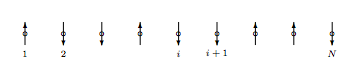$$\newcommand{\vecs}{\overset { \rightharpoonup} {\mathbf{#1}} }$$ $$\newcommand{\vecd}{\overset{-\!-\!\rightharpoonup}{\vphantom{a}\smash {#1}}}$$$$\newcommand{\id}{\mathrm{id}}$$ $$\newcommand{\Span}{\mathrm{span}}$$ $$\newcommand{\kernel}{\mathrm{null}\,}$$ $$\newcommand{\range}{\mathrm{range}\,}$$ $$\newcommand{\RealPart}{\mathrm{Re}}$$ $$\newcommand{\ImaginaryPart}{\mathrm{Im}}$$ $$\newcommand{\Argument}{\mathrm{Arg}}$$ $$\newcommand{\norm}{\| #1 \|}$$ $$\newcommand{\inner}{\langle #1, #2 \rangle}$$ $$\newcommand{\Span}{\mathrm{span}}$$ $$\newcommand{\id}{\mathrm{id}}$$ $$\newcommand{\Span}{\mathrm{span}}$$ $$\newcommand{\kernel}{\mathrm{null}\,}$$ $$\newcommand{\range}{\mathrm{range}\,}$$ $$\newcommand{\RealPart}{\mathrm{Re}}$$ $$\newcommand{\ImaginaryPart}{\mathrm{Im}}$$ $$\newcommand{\Argument}{\mathrm{Arg}}$$ $$\newcommand{\norm}{\| #1 \|}$$ $$\newcommand{\inner}{\langle #1, #2 \rangle}$$ $$\newcommand{\Span}{\mathrm{span}}$$$$\newcommand{\AA}{\unicode[.8,0]{x212B}}$$

2.25 Accessible configurations in another spin system

The Ising model for a ferromagnetic material such as nickel is different from the “ideal paramagnet” discussed in problem 2.9. In this model the spins reside at lattice sites and may point either up or down, but in contrast to the ideal paramagnet model, two adjacent spins do interact. Specifically, if two adjacent spins point in the same direction, the pair has energy $$−J$$. If they point in different directions, the pair has energy $$+J$$. (In the ferromagnetic situation considered here, $$J > 0$$.) We consider here a one-dimensional Ising model of $$N$$ sites with zero external magnetic field.a. Not every energy is possible for this model. What is the maximum possible energy? The minimum? What is the smallest possible energy spacing between configurations?

b. Consider the energy range from $$E$$ to $$E + ∆E$$ where $$∆E$$ is small compared to $$NJ$$ but large compared to $$J$$. What is the approximate number of states $$Ω(E, ∆E, J, N)$$ lying within this energy range?

c. Write down an expression for the entropy $$S(E, ∆E, J, N)$$ as a function of $$E$$. (Clue: Be careful to never take the logarithm of a number with dimensions.)

d. Take the thermodynamic limit to find the entropy per atom as a function of the energy per atom: $$s(e, J)$$. (Clue: Use Stirling’s approximation.)

e. Check your work by comparing the Ising entropy per spin $$s(e, J)$$ when the interactions vanish $$(J = 0)$$ to the ideal paramagnet entropy per spin $$s(e, H)$$ in zero field. (See problem 2.12. Clue: If $$J = 0$$, then $$e = 0$$ also. Why?)

General clues: It helps to concentrate not on the status of the spins (up or down) but on the status of the pairs (alike or different). How many nearest neighbor pairs are there? For each given configuration of pairs, how many corresponding configurations of spins are there?

2.26 Shake and spin

Make up a problem involving an ideal paramagnet with atoms free to move around.

2.27 Entropy as a function of mass

Use the Sackur-Tetrode formula to find the difference between the entropy of a sample of argon gas and a sample krypton gas under identical conditions. Use the data in Ihsan Barin, Thermochemical Data of Pure Substances, third edition (VCH Publishers, New York, 1995) to test this prediction at the temperatures 300 K and 2000 K.

2.28 (Q,D) For discussion: “The icy cold of outer space”

Is outer space at a high temperature or a low temperature? Before answering, consider these points:

a. The environment of outer space consists of protons and photons streaming away from the sun. The two systems are virtually uncoupled (do not interact with each other). I’m asking for the temperature of the protons.

b. It was implicitly (perhaps illicitly) assumed in section 2.7.3 that the centers of mass of the two systems in thermal contact were in the same reference frame. This is a general principle: When referring to the temperature of a moving body, we mean the temperature as measured by a thermometer moving along with that body.

c. Remember that in the arguments of section 2.7.3 the two systems could exchange energy but not particles.

d. What if we approximated the protons by an ideal gas?

e. Look at the Handbook of Chemistry and Physics under index entry “Temperature, Earth’s atmosphere”.

2.29 (Q,D) Errors in Reif

Scan Reif pages 47–61, but don’t read them in detail as they are rife with errors. Point out the major error in quantum mechanics made in each of the following passages. (Use a maximum of three sentences per passage.)

a. The examples on pages 48 and 49.

b. The second paragraph on page 57.

c. The first paragraph on page 60.

2.30 Polymers

I’d like to think up some problem concerning polymers of fixed length vs. polymers of fixed “activity for length”. The activity is analogous to chemical potential in that it is a control knob for polymer length. It might render the whole concept more concrete.

2.8: Additional Problems is shared under a not declared license and was authored, remixed, and/or curated by LibreTexts.